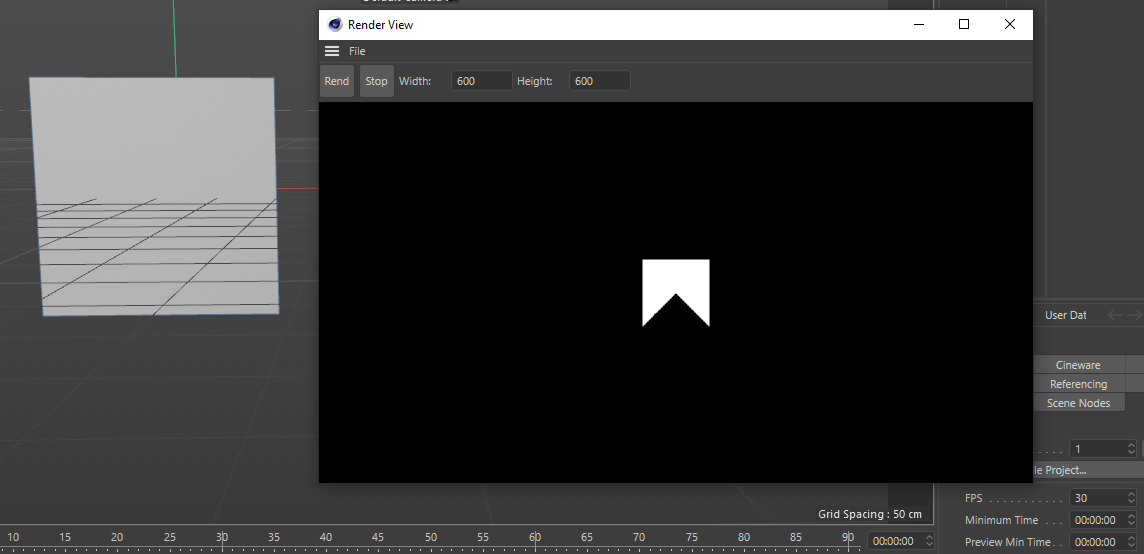# Solved Wrong Polygon indices

Hi everyone, I'm writing a small ray tracer, and I'm having problems with the mesh indices, the order is wrong.

``````for(int i = 0;i < meshes.size();i++)
{
for (int k = 0; k < meshes[i].PolyCount;k++)
{
Int32 indexPointA = meshes[i].indices[k].a;
Int32 indexPointB = meshes[i].indices[k].b;
Int32 indexPointC = meshes[i].indices[k].c;

Tri triangle;
triangle.vertex0 = (meshes[i].vertices[indexPointA]) / 100.0f;
triangle.vertex1 = (meshes[i].vertices[indexPointB]) / 100.0f;
triangle.vertex2 = (meshes[i].vertices[indexPointC]) / 100.0f;
IntersectTri(ray, triangle);
if (ray.t < 1e30f)
{
_film->setImagePixel(x, y, 255, 255, 255);
}
}
}
``````

I'm using a plane (previously triangulated) to test and get this result:i debuged the indices and they are wrong.
indices:
{1,3,0} - triangle 1
{1,2,3} - triangle 2

this is how i get the polygons:

``````void Scene::_recurseHierarchy(BaseObject* op)
{
while (op)
{
Bool isPolygon = op->IsInstanceOf(Opolygon) && !op->IsInstanceOf(Ojoint);
if (isPolygon)
{
Frog::Mesh mesh = {};
GePrint(op->GetName());

PolygonObject* poly = (PolygonObject*)op;
if (poly)
{
Int32 numOfVertices = poly->GetPointCount();
const CPolygon* cpoly = poly->GetPolygonR();
CPolygon* trianguled = nullptr;
const Vector* vetices = poly->GetPointR();
Int32 trianguledPolyCount;
Bool triRes = Triangulate(vetices, numOfVertices, &trianguled, &trianguledPolyCount);
if (triRes)
{
mesh.vertices = vetices;
mesh.indices = trianguled;
mesh.PolyCount = trianguledPolyCount;
objects.push_back(mesh);
}
}
}
_recurseHierarchy(op->GetDown());
op = op->GetNext();
}
}
``````

Can anyone point me what I'm doing wrong ?

Hi @fraukman2 thanks for posting, for me it's looks like a bug, since if you use a polygon primitive instead of a plane, it work as expected. I think there is a remapping needed for the point indices and polygon indices but I don't get it.

As it is the Christmas season, some members of the development team are away and the answer to this question may take longer than usual.
But I will keep you posted as soon as I have more information.

In the meantime, the easiest way would be to use MCOMMAND_TRIAGULATE that will triangulate the passed object.

``````BaseObject* op = doc->GetActiveObject();
if (!op)
return true;

// This will triangle op, if you dont want to affect the object in the scene call GetClone first.
PolygonObject* poly = static_cast<PolygonObject*>(op);

ModelingCommandData mcd;
mcd.op = op;
if (!SendModelingCommand(MCOMMAND_TRIANGULATE, mcd))
return false;

return true;
``````

Cheers,
Maxime.

MAXON SDK Specialist

Hi @fraukman2 thanks for posting, for me it's looks like a bug, since if you use a polygon primitive instead of a plane, it work as expected. I think there is a remapping needed for the point indices and polygon indices but I don't get it.

As it is the Christmas season, some members of the development team are away and the answer to this question may take longer than usual.
But I will keep you posted as soon as I have more information.

In the meantime, the easiest way would be to use MCOMMAND_TRIAGULATE that will triangulate the passed object.

``````BaseObject* op = doc->GetActiveObject();
if (!op)
return true;

// This will triangle op, if you dont want to affect the object in the scene call GetClone first.
PolygonObject* poly = static_cast<PolygonObject*>(op);

ModelingCommandData mcd;
mcd.op = op;
if (!SendModelingCommand(MCOMMAND_TRIANGULATE, mcd))
return false;